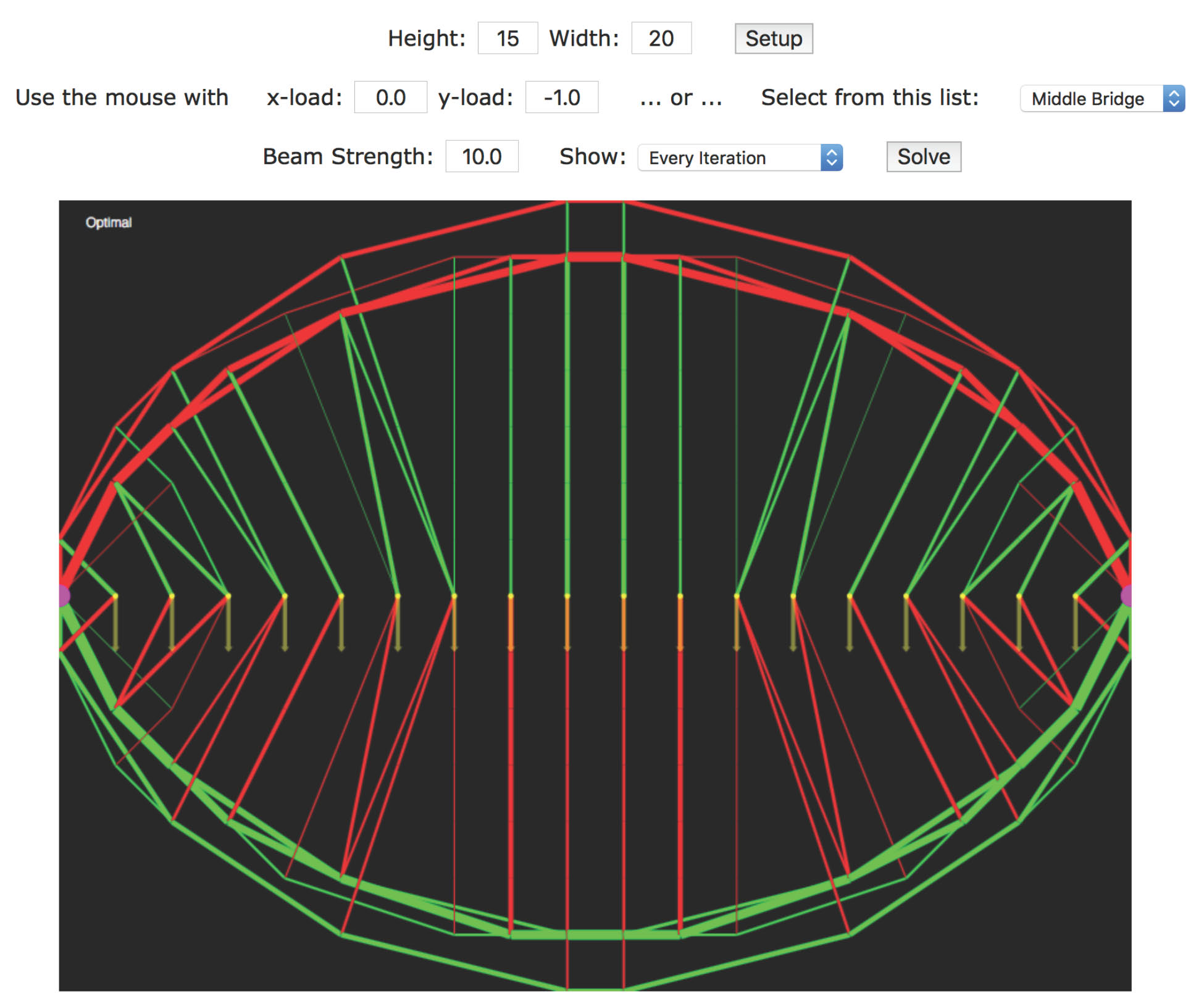## Algorithm Animation: Two-Phase Simplex Method

### via Structural Optimization

(Click here for older Java version.)

Height: Width:

Use the mouse with     x-load: y-load:     ... or ...     Select from this list:

Beam Strength:     Show:

#### Introduction

Since most people benefit greatly from geometric intuition, it is natural to try to represent a algorithms graphically. The obvious/textbook graphical representation of algorithms for linear programming involve restricting ones attention to situations with at most 3 variables. However, real-world linear programming problems involve thousands of variables and constraints. It would be nice to have graphical representations for these problems too. If one is willing to restrict ones attention to a specific application area, then it is often easy to come up with a nice representation of the problem.

#### Minimum-Weight Structural Design

Here we consider the minimum-weight structural design problem. A simple version of this problem is defined as follows. One starts with a graph (i.e., list of nodes and connecting arcs) that represents places in 2 or 3 dimensional space where structural beams could be placed. The problem is to design a structure that is anchored at certain specified nodes and that can support a specified load (i.e., force vector) at certain other specific nodes. We assume that each beam must have a cross sectional area that is proportional to the tension/compression that that beam must carry in order to have force balance at every node in the structure. Of course, the objective is to minimize weight, which is assumed to be proportional to the sum of the volumes of the beams. Without going into the details (see Chapter 15 of Linear Programming: Foundations and Extensions), the problem can be easily formulated as a linear programming problem.

#### Two-Phase Simplex Algorithm

The applet below animates the two-phase simplex algorithm for solving linear programming problems. Details of the two-phase simplex method can be found in Chapter 6 of Linear Programming: Foundations and Extensions).

#### Running the Animation

The graph on which the design takes place is a rectangular lattice of nodes with each node connected by an arc to its 8 nearest neighbors, the nearest neighbors of them and the nearest neighbors yet again of those. To set up a problem, first select the dimensions for the rectangular lattice (i.e., the height and the width). Then click on the Setup button. In setup mode, whenever you click near a node, that node gets the load vector currently displayed in the x-load and y-load textfields. The load vectors are not actually shown graphically other than simply to highlight the node in yellow. Clicking near a node while holding down the shift key tells the applet that that node is to be an anchor node. Generally speaking, you'll need at least two anchor nodes in order to design a stationary structure. Anchor nodes are highlighted in magenta.

After setting the load and anchor nodes, click on solve to start the animation.

In the optimal structure, beams with zero cross-sectional area are, of course, simply not built. Of the beams that remain, some are under compression and others are under tension. The compressed beams are shown in red while the beams under tension are shown in green. As the algorithm progresses from an initial solution to the optimal solution, there are times when beams one or both of the tension/compression components of the force are given negative values. These ``negative'' beams are shown in magenta and teal.

There are a few parameters that you can play with. You can select whether you want to see an update of the animation on every simplex pivot or just once every 200 pivots. Usually, the animation looks best if updated with every pivot.

The second parameter is the strength of the beam material. A larger value gives smaller cross sections. You should simply adjust this parameter as appropriate to get a nice visual representation for each specific problem.

Here's an example: